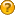###Author Topic: Money Equation for LIfe  (Read 1659 times)

#### santoshlv426##### Money Equation for LIfe
« on: December 30, 2015, 08:47:44 am »
Hi guys,
Been some time since  I've been to the site.

My question : Is there, or has there been a mathematical equation derived to answer "how much do I need to stop working right now ?", with known inputs viz.

1. Available Capital (C)
2. Annual Draw-down (D)
3. Interest Growth rate of invested capital (i)
4. Time (T - this would typically be e.g (75 - Age today)

Thus : R (Required Capital) = *F(C,D,i,T)

Such an equation could be used or rearranged to solve for required Interest Rate (i) if a certain Capital (R) is available to invest, thus i = *F(R,C,D,T)

If such an equation is available, can one of you fine gentlemen share this with me or point me to the text where such statistical analysis is undertaken and hopefully something has been published. As an aside, I've tried to do some high level modelling for the above two equations and it "seems" that this can be described using a 4th order Polynomial equation.
Would this be on the right track ?

#### czc##### Re: Money Equation for LIfe
« Reply #1 on: December 31, 2015, 05:45:02 pm »

No complex equations but it seems to work. Just need to confirm the workability for this country.

#### Patrick

• Hero Member
••• Posts: 2485
• Karma: +43/-2##### Re: Money Equation for LIfe
« Reply #2 on: January 04, 2016, 07:43:07 am »